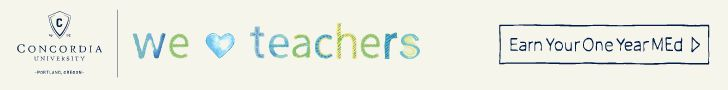# Kindergarten Mathematics StandardsA kindergartner will learn to count, to read and write numbers up to 20 and simple addition and subtraction. Here are a few examples of what your child will learn and practice throughout the year, questions to ask his or her teachers and tips for reinforcing academic skills at home.

### Counting and Cardinality

1. Count by ones and tens to 100.
Ones: 1, 2, 3, 4, 5, 6, 7, 8, 9, 10, 11, …, 97, 98, 99, 100
Tens: 10, 20, 30, 40, 50, 60, 70, 80, 90, 100
2. Compare numbers between 1 and 10; be able to choose the bigger number.
Q: Which number is bigger, 5 or 9?
A: 9 is bigger
3. Count objects in a group up to 20; compare two groups and tell whether they are equal or if one group is greater than or less than the other.
Q: You have a group of 10 objects and another group of 15 objects. Which one is bigger?
A: The group of 15 objects is bigger.

### Operations and Algebraic Thinking

1. Understand addition and subtraction as “adding to” and “taking away,” respectively.
5 take away 3 = 5 – 3 = 2
2. Depict adding and subtracting using objects, shapes, fingers, drawings and sounds (such as snapping or clapping).
3. Using the numbers 1 through 9, find two numbers that add up to 10.
9 + 1 = 10
8 + 2 = 10
7 + 3 = 10
6 + 4 = 10
5 + 5 = 10
4. Add and subtract within 5 quickly and easily.

### Number and Operations in Base Ten

1. Recognize numbers 11-19 as ten plus additional ones.
10 + 1 = 11
10 + 2 = 12

10 + 9 = 19

### Measurement and Data

1. Understand the different ways objects can be measured such as length, height and weight (how long, how tall, how heavy).

### Geometry

1. Name shapes and use simple language to describe them.
Triangles have three sides and circles are round.
Learn More: Click to view related resources.